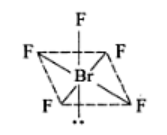# Using vsper theory draw the shape of PCl5 and BrF5

using vsper theory draw the shape of PCl5 and BrF5

PCl5: sp3d hybridization having triagonal bipyramid shape with five 3sp3d-3p bonds.
The valence electrons in PCl5 = 5 + 5 × 7 = 40. The distribution of these electrons in PCl5 is as follows.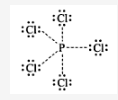There are five lone pairs of electrons around P. Hence, the arrangement of these electrons around P will be triagonal bipyramid, The hybridization involved in this complex is sp3d.

Hence the shape of PCl5 is: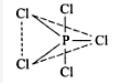BrF5: sp3d2 hybradization having octahedral shape with one position occupied by lone pair (or square pyramidal) with five 4sp3d2-2p bonds.

The valance electrons in BrF5 = 7 + 5 × 7 = 42. The distribution of these electrons in BrF5 is as follows.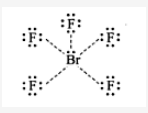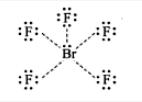There are six lone pairs of electrons around Br. Hence, the arrangement of these electrons around Br will be octahedral; The hybridization involved in this complex is sp3d2.

Hence the shape BrF5 is: